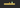# Conditional rendering in React with examples

Home » react » Conditional rendering in React with examplesIn this tutorial, we are going to learn about what is conditional rendering and how to implement it in react.

## What is conditional rendering?

Conditional rendering means only display the elements in the UI if the particular condition is true or false.

### IF/ELSE conditionals

Let’s look into JavaScript if-else conditionals.

``````let num = 2

if(num === 2){
console.log('the given number is 2')
}else{
console.log('the give number is not 2')
}``````

In the above code, we have initialized a variable with 2 then we used if else conditional to log the message based on the provided number.

Let’s implement the if/else conditional in react.js.

``````function App(props){

if(props.num === 2){
return <p>The given number is 2</p>
}else{
return <p>The given number is not  2</p>
}

}

<App  num = {2} />``````

In the `App` component we passed `num prop` to 2 so that we can only render the `p` element present inside the if condition.

### Ternary operator

We can make our App component code shorter by using the ternary operator.

``````function App(props){

{props.num === 2 ?  <p>The given number is 2</p> :
<p>The given number is not 2</p> }

}

<App  num = {2} />``````

Here we wrapped our code with curly braces because in react jsx we need to use curly braces for the JavaScript expressions.

### Logical &&(and) operator

In JavaScript, the Logical && operator is used to evaluates the expression if the given condition is true.

``````true && expression // expression

false && expression // false``````

``````function Search(props){

return (
<div>
<h1>Search</h1>
<button>Search</button>

{props.searchResults > 0 &&  <ul>search results</ul>}

</div>
)

}``````

In the `Search` component we used Logical &&(and) operator to render the `ul` element only if `props.results` is greater than 0.